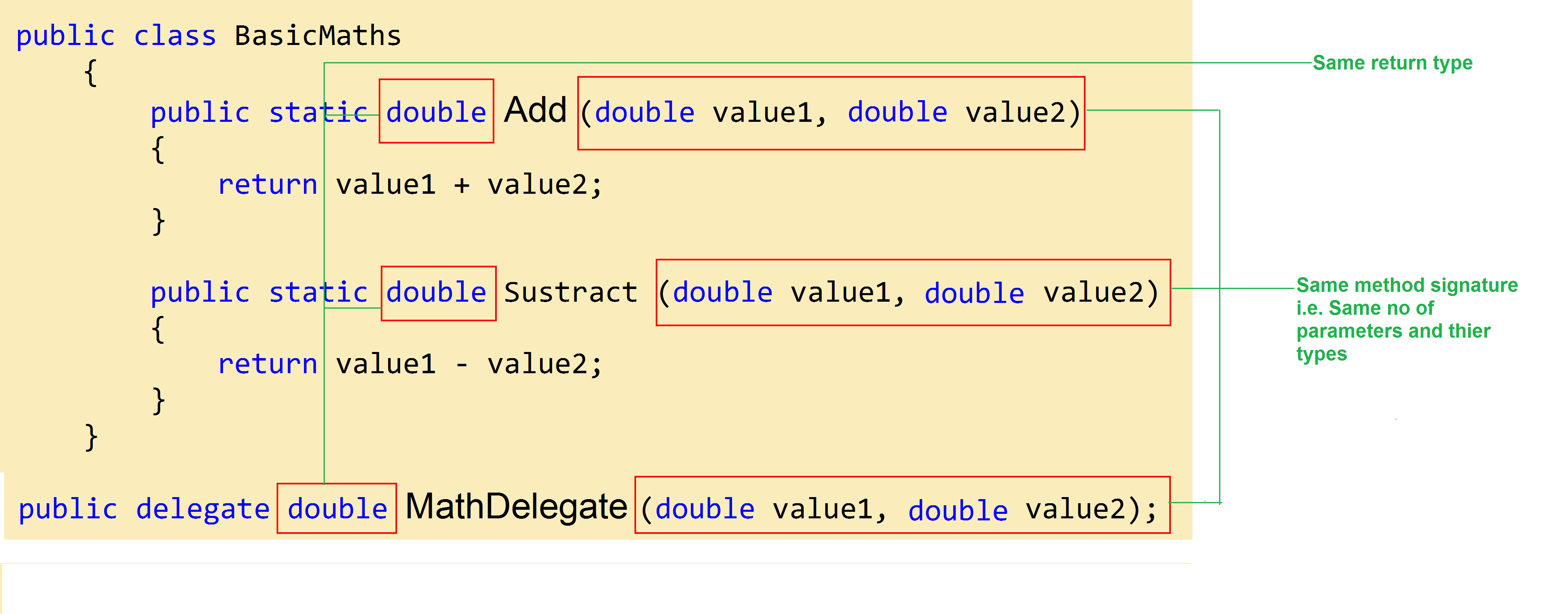# Back To Basics – Delegate in C#

By | May 6, 2015A delegate person is someone who represent an organization or set of people. In C# world a delegate is a reference type variable that represent one or set of methods by holding there reference.

Note: The reference can be changed at runtime.  So let’s first understand what it meant.

Let’s have a class called BasicMaths having two methods Add and Subtract, which performs addition and subtraction of two numbers respectively.

```public class BasicMaths
{
public static double Add (double value1, double value2)
{
return value1 + value2;
}

public static double Subtract (double value1, double value2)
{
return value1 - value2;
}
}
```

The first point one should keep in mind that a Delegate is type safe which means it can only hold reference of methods which have the same numbers of parameters, types and return type, which mean in our case as both methods have two parameters, both of type double and the return type is also same i.e. Double, hence it be referenced by a Delegate that has same skeleton, let’s declare our Delegate.

```public delegate double MathDelegate (double value1, double value2);
```

Note: it looks similar to the methods Add and Subtract, let’s have a closer look.Now let’s concentrate on how to invoke a delegate. In order to do so first we need to create an instance of the delegate and register method to it, it can be at the time of instantiating the delegate as shown below:

```public class MathTest
{
public delegate double MathDelegate (double value1, double value2);
public static void Main()
{
var result = mathDelegate(5, 2);
Console.WriteLine(result);

// output: 7

&nbsp;

MathDelegate anotherMathDelegate = new MathDelegate(BasicMaths.Subtract);
result = anotherMathDelegate(5, 2);
Console.WriteLine(result);

// output: 3
}
}
```

Multicasting of a Delegate

We can create an invocation list of methods which can be called when a delegate is invoked, this is called multicasting of a delegate.

In order to do so we need to be aware of role of + and – operator. The + operator helps in adding items (method reference) to the delegate invocation list while the minus operator helps in removing items from the invocation list.

Let’s see how it works:

```public class Test
{
public delegate double MathDelegate (double value1, double value2);
public static void Main()
{
mathDelegate += BasicMaths.Subtract;

// now when mathDelegate is invoked it calls both the method BasicMaths.Add and BasicMaths. Subtract.

// if we wants BasicMaths.Add to be removed from invocation list we can do

// so that next time when the delegate is invoke it only calls BasicMaths.Subtract
}
}

```

Note:

1. The sequence in which the methods are called are same in which they are added in the invocation list.
2. It’s not necessary that delegates only hold a reference to static methods.

Usage of Delegates

Let’s have a closer look into real time usage of delegates.

Suppose we want to have a situation when a user wants to print an item it gets printed on more than one place for the sake of simplicity, let’s assume the results to be printed on console application as well as a flat file.

This can be done as, at each time when you want to print either call methods to print on console as well as writing on flat file individually or you can create a delegate which holds reference to these methods and just invoke the delegate when you want to print, we are going to do it in the second way using delegates.

```
public class ConsolePrinting
{
public void WriteToConsole(int number)
{
Console.WriteLine("The number is - {0}", number);
}
}

public class FilePrinting
{
public void PrintIntoFile(int number)
{
using (var fileStream = new FileStream(@"c:\demo\file.txt", FileMode.Append, FileAccess.Write))
{
using (var streamWriter = new StreamWriter(fileStream))
{
streamWriter.WriteLine("The number is - {0}", number);
}
}
}
}

public class MulticastDeletage
{
public delegate void Printing(int number);
public static void Main()
{
var filePrinting = new FilePrinting();
var consolePrinting = new ConsolePrinting();
var printing = new Printing(filePrinting.PrintIntoFile);
printing += consolePrinting.WriteToConsole;
for (int i = 1; i &lt; 11; i++)
{
if (i % 2 == 0)
{
printing(i);
}
}
}
}

```Author: Shashank Bisen

Shashank Bisen is curious blogger and speaker who love to share his knowledge and experience which can help anyone in their day to day work.

## 7 thoughts on “Back To Basics – Delegate in C#”

1.Manish

Really nice article. Exactly matching to the title of article. Keep posting in the community with these titles.

2.Christoph

I think there is a mistake in the code of Multicasting of a delegate.
// if we wants BasicMaths.Add to be removed from invocation list we can do
3.Shashank Bisen Post author
4.Dan